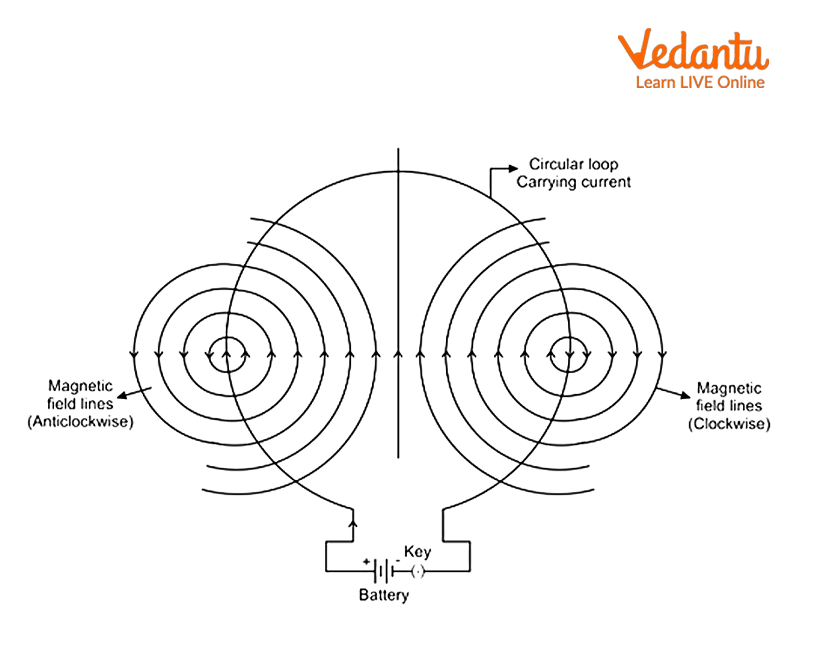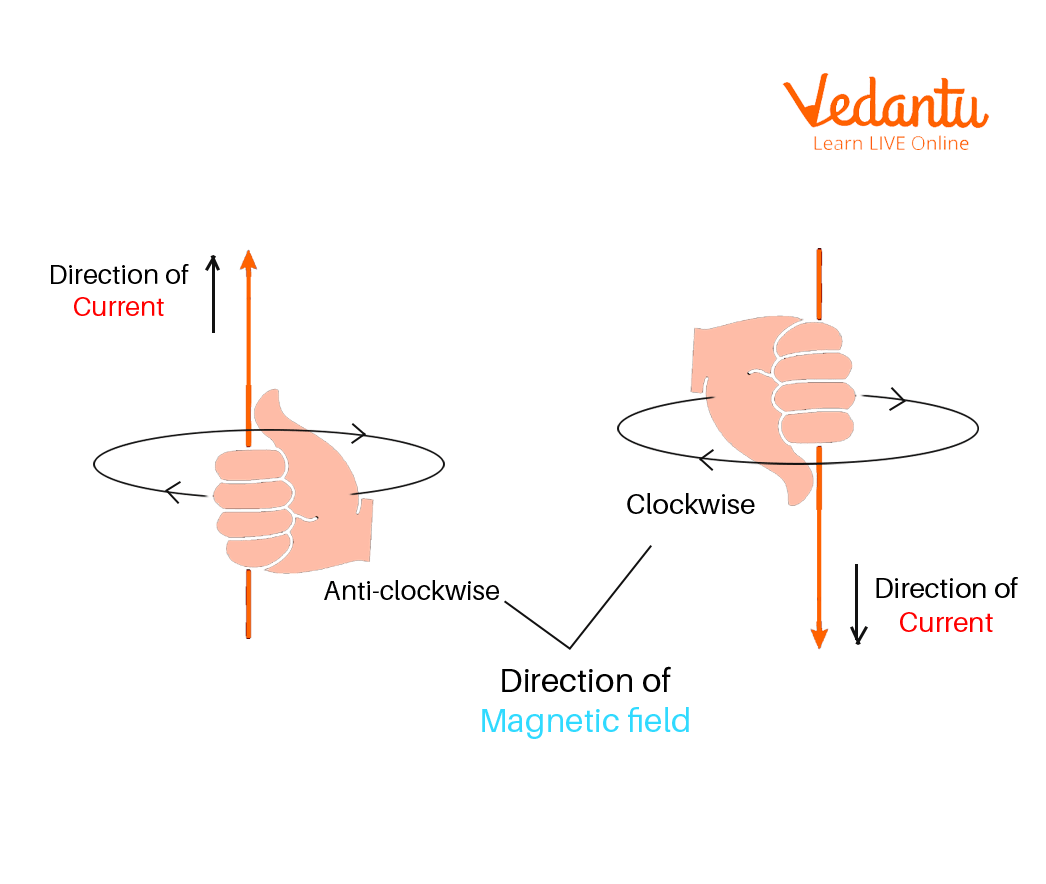Courses
Courses for Kids
Free study material
Offline Centres
More

# Magnetic Field Due to a Current Through a Circular LoopLast updated date: 27th Nov 2023
Total views: 117.9k
Views today: 4.17k## A Brief Overview of Magnetic Field

With the help of magnetic field lines, we can visualise the magnetic field. The pattern of the magnetic field lines depends on the shape of the magnet. The magnetic field lines give us the pattern of the magnetic field. Using iron filings or a magnetic compass, we can find the shape of the magnetic field.

In this article, we will get a deep insight into the magnetic field produced by a current carrying circular loop and Maxwell’s right hand thumb rule to find the direction of the magnetic field.

## History of Magnetism

Magnetism has been known since ancient times. In 1820, Hans Christian Oersted invented a very useful phenomenon. He was born in Rudkobing, Denmark. He observed that when the electric wire carries electric current, it behaves like a magnet. He established the relation between electricity and magnetism in the 19th Century. He played a crucial role in understanding electromagnetism.

## What is a Magnetic Field?

The region surrounding the magnet in which the force of the magnet can be felt is called the extent of the magnetic field. Its SI unit is Tesla and it is named after the American Scientist Nikola Tesla. Gauss is the smaller unit of the magnetic field.

Magnetic field lines generally originate from the North Pole of the magnet and end at the South Pole but inside the magnet, magnetic field lines are directed from the South Pole to the North Pole. Thus, we can say that magnetic field lines form a closed curve. They can never intersect with each other.

## Magnetic Field Due to Current Carrying Circular Loop

The magnetic field lines due to current through a circular loop is shown in the figure given below.Magnetic Field Due to Current Carrying Circular Loop

Circular loop is a collection of many straight lines. Here, one end of a circular loop is connected to a positive end of a battery and another end is connected with the negative end of the battery. So, current can flow and in the circular loop, it is moving in clockwise direction as shown in above figure. We know that when current flows in a conductor, the magnetic field will get generated which can produce magnetic field lines.

We can observe that the magnetic field lines are in the form of concentric circles near the sides of the loop but towards the centre of the loop, the magnetic field lines become almost parallel which indicates that this part of the coil has a uniform magnetic field. In other words, we can say that at the centre of the circular loop, magnetic field lines become straight lines. At every point of a loop, the concentric circles representing the magnetic field around it would become larger and larger as we move away from the loop. The strength increases as we go closer to the centre of the loop.

In the case of the straight current carrying wire, if we know the direction of current, we can find the direction of the magnetic field using Maxwell’s right hand thumb rule. This rule can be applied for finding the direction of magnetic field of this current carrying loop as well.Maxwell’s Right Hand Thumb Rule

This rule says that if your thumb represents the direction of current, then the direction in which all other four fingers are curled represent the direction of the magnetic field. So, in order to apply the right-hand thumb rule, hold a loop in your right hand such that your thumb points the direction of current in this loop, then the direction in which fingers are wrapped around the loop is the direction of the magnetic field. This is shown in the above figure.

By applying this rule, we find that each small section of the circular loop contributes to the magnetic field lines in the same direction within the loop. This is how a current carrying loop behaves.

The magnetic field intensity due to current carrying circular loop (at the centre) is given by,

$B = \dfrac{{{\mu _0}NI}}{{2r}}$

Where, ${\mu _0} = 4\pi \times {10^{ - 7}}Tm{A^{ - 1}}$ and it is the permeability of free space

$I$ is the current flowing in the loop

$r$ is the radius of the loop

$N$ is the number of turns of the loop

## Factors on Which the Magnetic Field Produced by a Current Carrying Circular Loop Depend

The magnetic field produced by this current carrying circular loop at a given point is

• Directly proportional to the amount of current flowing through it.

This means B is proportional to I

• Directly proportional to the number of turns of the loop.

This means N is proportional to I

This happens because the current in each turn would be in the same direction. Hence, the field due to these adds up.

• Inversely proportional to the radius of the loop.

This means B is inversely proportional to 1/r

## Solved Examples

1. A tightly wound 100 turn coil of radius $10cm$ is carrying a current of $1A$

Find the value of the magnetic field at the centre of the coil.

Ans: Given: Radius of the coil is $r = 10cm = 10 \times {10^{ - 2}} = 0.1m$

$I = 1A$

${\mu _0} = 4\pi \times {10^{ - 7}}Tm{A^{ - 1}}$ is the permeability of free

space

Number of turns $N = 100$

The value of the magnetic field at the centre of the coil is given by,

$B = \dfrac{{{\mu _0}}}{2}\dfrac{{NI}}{r}$

Substituting the given values in above equation,

We will get, $B = \dfrac{{4\pi \times {{10}^{ - 7}}}}{2}\dfrac{{100 \times 1}}{{0.1}}$

$\therefore B = 6.28 \times {10^{ - 4}}T$

So, the value of the magnetic field is $6.28 \times {10^{ - 4}}T$

1. An $\alpha$ particle is completing one circular round of radius $0.8m$ in 2 seconds. Find the magnetic field at the centre of the circle. (Given: electronic charge $e = 1.6 \times {10^{ - 19}}C$ )

Ans: Given: Radius of circle is $r = 0.8m$

Time taken is $t = 2\sec$

We know that ${\mu _0} = 4\pi \times {10^{ - 7}}Tm{A^{ - 1}}$ is the permeability of free space

The charge $q$ on $\alpha$ particle is $2e = 2 \times \left( {1.6 \times {{10}^{ - 19}}} \right) = 3.2 \times {10^{ - 19}}$

The amount of charge which flows through any conductor per unit time is known as current and we can express it by $I = \dfrac{q}{t} = \dfrac{{2e}}{t}$

Substituting the values in above equation,

We will get, $I = \dfrac{{3.2 \times {{10}^{ - 19}}}}{2} = 1.6 \times {10^{ - 19}}A$

Magnetic field at the centre of the circle is given by, $B = \dfrac{{{\mu _0}I}}{{2r}}$

Substituting the values in above equation,

We will get, $B = \dfrac{{\left( {4\pi \times {{10}^{ - 7}}} \right) \times \left( {1.6 \times {{10}^{ - 19}}} \right)}}{{2 \times 0.8}} = 12.57 \times {10^{26}}T$

The value of magnetic field at the centre of the circle is $12.57 \times {10^{ - 26}}T$

## Interesting Facts

• The South Pole of the Earth’s magnet is in the geographical North and the North Pole of the Earth's magnet is in the geographical South.

• Magnetite is the most magnetic natural metal on the Earth.

• Magnets generate a non-contact force. The force which acts without physical contact between two objects is called non-contact force. In this type of force, physical contact is not required.

## Conclusion

From the above discussion, we can conclude that when we reach the centre of the loop, the magnetic field at the centre of a circular coil appears to be straight line. The strength of the magnetic field decreases with an increase in distance from the loop. We have also discussed the factors affecting the magnetic field produced by a current carrying circular loop and some interesting facts. We hope to have helped you with your queries and doubts.

## FAQs on Magnetic Field Due to a Current Through a Circular Loop

1. State the difference between electromagnets and permanent magnets.

Permanent magnets are the regular magnets. In permanent magnets, the magnetism is permanent unless damaged. Permanent magnets are used in fridge magnets, toys, microphones, loudspeakers and other devices. Permanent magnets are made of steel and alloys such as carbon steel, chromium steel or alnico which is an alloy of aluminium, nickel, cobalt and iron are used.

Electromagnets are temporary and are made using a long insulated copper wire wrapped around a soft iron core. Current is passed through this copper wire. Electromagnets are used in electric bells, maglev trains and in MRI machines.

2. Explain properties of diamagnetic substances.

Here are some properties of diamagnetic substances:

• Diamagnetic substances are repelled by a magnet. Copper, bismuth, diamond, quartz and mercury are some examples of diamagnetic substances.

• Diamagnetic materials are magnetised feebly in the opposite direction to the applied external field.

• The atoms of the diamagnetic substances do not have the resultant magnetic moment.

• When suspended in a uniform magnetic field, it comes to rest in the perpendicular direction to the magnetic field.

• When it is kept in a non uniform magnetic field, it moves from the region of greater field to the region of less field strength.

• Diamagnetism does not depend upon temperature.

3. State the differences between a bar magnet and a solenoid.

Here are a few points which shows the difference between them:

• In a bar magnet, magnetic poles can not be changed. This means that polarity is fixed and can not be easily reversed. The magnetic poles in solenoid can be interchanged. In other words, we can say that polarity can be reversed by changing the direction of current in the case of the solenoid.

• The magnetism is permanent in the bar magnet. It is temporary in the solenoid.

• Another difference is that the strength of the magnetic field remains the same in the bar magnet. We can either increase or decrease the magnetic field strength in the solenoid.